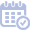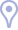# Talks

Topics in Minimum-Mean-Squared-Error (MMSE) Estimation in Sparse ApproximationJune 10th, 2010Sparsity and Computation workshop, Bonn.

Among the many ways to model signals, a recent approach that draws considerable attention is sparse representation modeling. In this model, the signal is assumed to be generated as a random linear combination of a few atoms from a pre-specified dictionary. In this talk we describe our recent work that analyzed two Bayesian denoising algorithms — the Maximum-Aposteriori Probability (MAP) and the Minimum-Mean-Squared-Error (MMSE) estimators, under the assumption that the dictionary is unitary. It is well known that both these estimators lead to a scalar shrinkage on the transformed coefficients, albeit with a different response curve. In this talk we start by deriving closed-form expressions for these shrinkage curves and then analyze their performance. Upper bounds on the MAP and the MMSE estimation errors are derived. We tie these to the error obtained by a so-called oracle estimator, where the support is given, establishing a worst-case gain-factor between the MAP/MMSE estimation errors and the oracle’s performance.This is a joint work with Irad Yavneh, Javier Turek, and Matan Protter (CS - Technion).